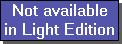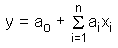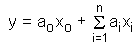The SDL Component Suite is an industry leading collection of components supporting scientific and engineering computing. Please visit the SDL Web site for more information....## MultiLinRegUnit: SDL_math2 Class: none Declaration: function MultiLinReg (InData: TMatrix; OutData, Coeff: TVector; DeltaCoeff: TVector; var NearSingular: boolean): boolean;

The function MultiLinReg calculates the best approximated solution for an overdetermined equation system (more equations than variables). The matrix InData contains the independent variables xi, the vector OutData contains the values of the dependent variable y. The function returns a TRUE value if the result is valid. In this case the coefficients a1 to an of the solution are contained in vector Coeff. Numerical instabilities which may arise from near-singular equations are indicated by returning a TRUE value in the variable parameter NearSingular. In this case the calculated coefficients should not be used.

The vector DeltaCoeff reflects the uncertainties of the estimated parameters in vector Coeff. In order to get the standard deviation of the parameters, DeltaCoeff has to be multiplied by the standard error of the residuals. The standard error can be calculated bywith n = number of rows of InData, k = number of columns of InData, and the Yi being the actual and the estimated OutData.

The equation system to be solved can be denoted as follows (the coefficients ai correspond to the parameter Coeff, the values yi are stored in OutData, and the values xij are stored in InData ):Please note that most often models involve a constant term, i.e.In order to find a general least squares solution for such systems, you have to formally extend the equation towith x0 = 1. This means that such models can be calculated by adding an extra column to the input data InData, filling this extra column by a constant value of 1.0. The vectors Coeff and DeltaCoeff have to be enlarged by 1 element accordingly.

 Hint 1: MultiLinReg uses singular value decomposition to obtain the solution.

 Hint 2: If the number of variables n is equal to the number of observations m MultiLinReg calculates the exact solution.

 Hint 3: The contents of the matrix InData will be changed by the method MultiLinReg. If you need the data in matrix InData later-on you have to create a copy of the matrix before applying MultiLinReg.

 Example: This property is used in the following example program (see http://www.lohninger.com/examples.html for downloading the code): multilinreg

Last Update: 2013-May-30